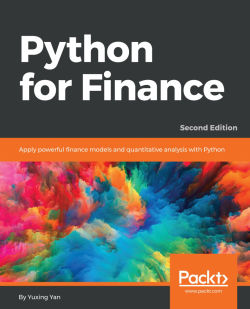# Python for Finance - Second Edition3.6 (5 reviews total)
By Yuxing Yan

• Constantly updated with 100+ new titles each month
• Breadth and depth in over 1,000+ technologies

This book uses Python as its computational tool. Since Python is free, any school or organization can download and use it.

This book is organized according to various finance subjects. In other words, the first edition focuses more on Python, while the second edition is truly trying to apply Python to finance.

The book starts by explaining topics exclusively related to Python. Then we deal with critical parts of Python, explaining concepts such as time value of money stock and bond evaluations, capital asset pricing model, multi-factor models, time series analysis, portfolio theory, options and futures.

This book will help us to learn or review the basics of quantitative finance and apply Python to solve various problems, such as estimating IBM’s market risk, running a Fama-French 3-factor, 5-factor, or Fama-French-Carhart 4 factor model, estimating the VaR of a 5-stock portfolio, estimating the optimal portfolio, and constructing the efficient frontier for a 20-stock portfolio with real-world stock, and with Monte Carlo Simulation. Later, we will also learn how to replicate the famous Black-Scholes-Merton option model and how to price exotic options such as the average price call option.

Publication date:
June 2017
Publisher
Packt
Pages
586
ISBN
9781787125698

## Chapter 1. Python Basics

In this chapter, we will discuss basic concepts and several widely used functions related to Python. This chapter plus the next one (Chapter 2, Introduction to Python Modules) are only the chapters exclusively based on Python techniques. Those two chapters serve as a review for readers who have some basic Python knowledge. There is no way that a beginner, with no prior Python knowledge, could master Python by reading just those two chapters. For a new learner who wants to learn Python in more detail, he/she could find many good books. From Chapter 3, Time Value of Money onward, we will use Python, which will help in explaining or demonstrating various finance concepts, running regression, and processing data related to economics, finance, and accounting. Because of this, we will offer more Python-related techniques and usages in each of the upcoming chapters.

In particular, in this chapter, we will discuss the following topics:

• Python installation

• Variable assignment, empty space, and writing our own programs

• Writing a Python function

• Data input

• Data manipulation

• Data output

## Python installation

In this section, we will discuss how to install Python. More specifically, we will discuss two methods: installing Python via Anaconda and installing Python directly.

There are several reasons why the first method is preferred:

• First, we can use a Python editor called Spyder, which is quite convenient for writing and editing our Python programs. For example, it has several windows (panels): one for the console, where we can type our commands directly; one for the program editor, where we can write and edit our programs; one for Variable Explorer,where we can view our variables and their values; and one for help, where we can seek help.

• Second, different colors for codes or comment lines will help us avoid some obvious typos and mistakes.

• Third, when installing Anaconda, many modules are installed simultaneously. A module is a set of programs written by experts, professionals, or any person around a specific topic. It could be viewed as a toolbox for a specific task. To speed up the process of developing new tools, a new module usually depends on the functions embedded in other, already developed modules. This is called module dependency. One disadvantage of such a module dependency is how to install them at the same time. For more information about this, see Chapter 2, Introduction to Python Modules.

### Installation of Python via Anaconda

We could install Python in several ways. The consequence is that we will have different environments for writing a Python program and running a Python program.

The following is a simple two-step approach. First, we go to http://continuum.io/downloads and find an appropriate package; see the following screenshot: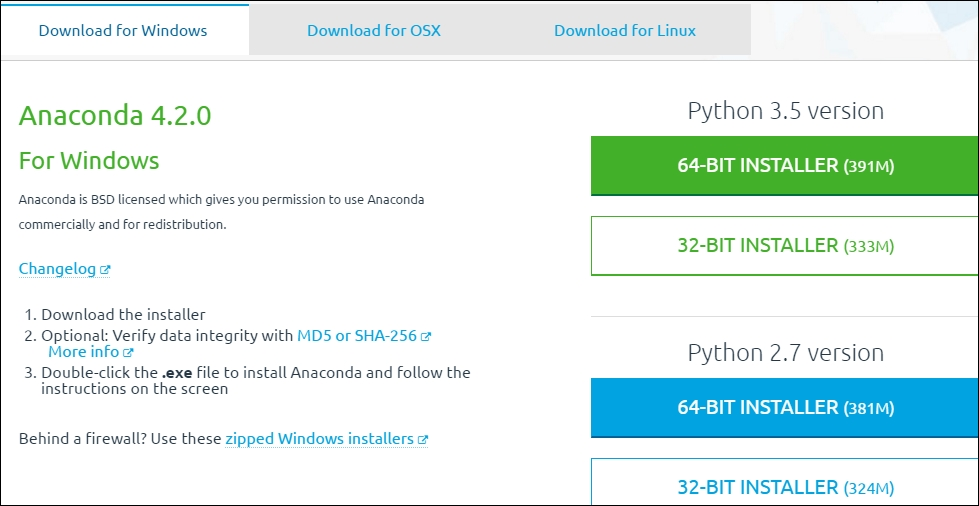For Python, different versions coexist. From the preceding screenshot, we see that there exist two versions, 3.5 and 2.7.

For this book, the version is not that critical. The old version had fewer problems while the new one usually has new improvements. Again, module dependency could be a big headache; see Chapter 2, Introduction to Python Modules for more detail. The version of Anaconda is 4.2.0. Since we will launch Python through Spyder, it might have different versions as well.

### Launching Python via Spyder

After Python is installed via Anaconda, we can navigate to Start (for a Windows version) |All Programs |Anaconda3(32-bit), as shown in the following screenshot: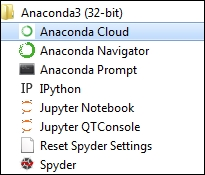After we click Spyder, the last entry in the preceding screenshot, we will see the following four panels: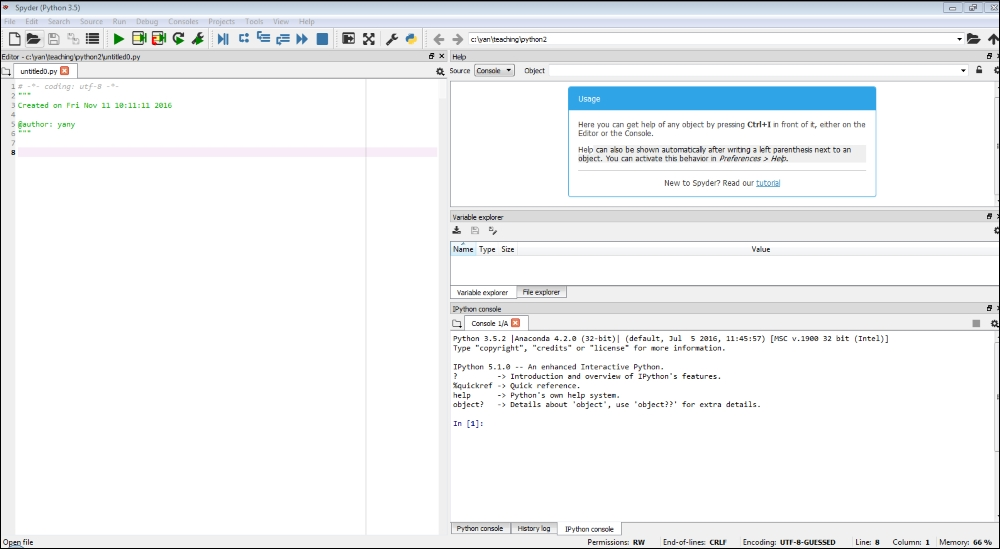The top-left panel (window) is our program editor, where we write our programs. The bottom-right panel is the IPython console, where we cantype our simple commands. IPython is the default one. To know more about IPython, just type a question mark; see the following screenshot: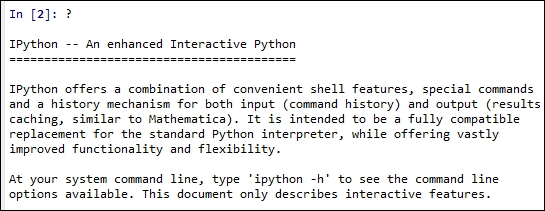Alternatively, we could launch Python console by clicking Consoles on the menu bar and then Open a Python console. After that, the following window will appear: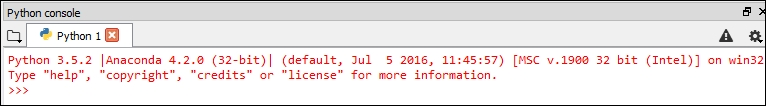From the image with four panels, the top-right panel is our help window, where we can seek help. The middle one is called Variable Explorer, where the names of variables and their values are shown. Depending on personal preference, users will scale those panels or reorganize them.

### Direct installation of Python

For most users, knowing how to install Python via Anaconda is more than enough. Just for completeness, here the second way to install Python is presented.

The following steps are involved:2. Depending on your computer, choose the appropriate package, for example, Python version 3.5.2. For this book, the version of Python is not important. At this stage, a new user could just install Python with the latest version. After installation, we will see the following entries for a Windows version: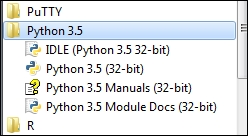3. To launch Python, we could click `IDLE (Python 3.5. 32 bit)` and get to see the following screen: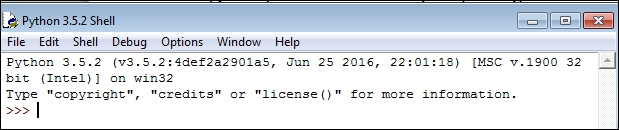4. From the IPython shown in the screenshot with four panels, or from the Python console panel or from the previous screenshot showing Python Shell, we could type various commands, as shown here:

```>>>pv=100
>>>pv*(1+0.1)**20
672.7499949325611
>>> import math
>>>math.sqrt(3)
1.7320508075688772
>>>
```
5. To write a Python program, we click File, then New File: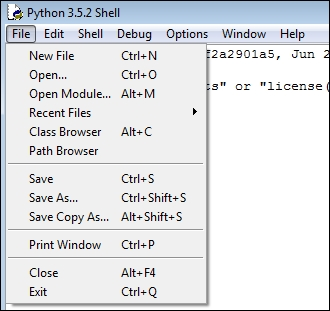6. Type this program and then save it: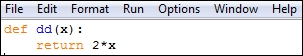7. Click Run, then Run module. If no error occurs, we can use the function just like other embedded functions, as shown here: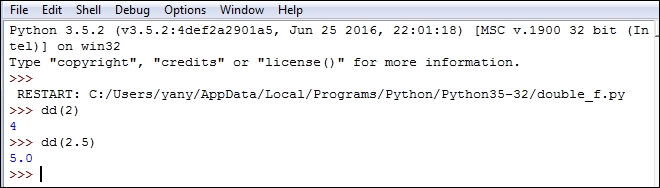## Variable assignment, empty space, and writing our own programs

First, for Python language, an empty space or spaces is very important. For example, if we accidently have a space before typing `pv=100`, we will see the following error message: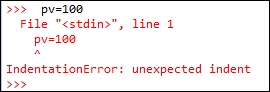The name of the error is called `IndentationError`. The reason is that, for Python, indentation is important. Later in the chapter, we will learn that a proper indentation will regulate/define how we write a function or why a group of codes belongs to a specific topic, function, or loop.

Assume that we deposit \$100 in the bank today. What will be the value 3 years later if the bank offers us an annual deposit rate of 1.5%? The related codes is shown here:

```>>>pv=100
>>>pv
100
>>>pv*(1+0.015)**3
104.56783749999997
>>>```

In the preceding codes, `**` means a power function. For example, `2**3` has a value of `8`. To view the value of a variable, we simply type its name; see the previous example. The formula used is given here: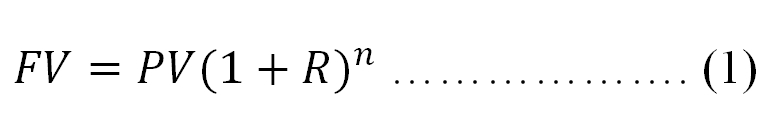Here, FV is the future value, PV is the present value, R is the period deposit rate while n is the number of periods. In this case, R is the annual rate of 0.015 while n is 3. At the moment, readers should focus on simple Python concepts and operations.

In Chapter 3, Time Value of Money, this formula will be explained in detail. Since Python is case-sensitive, an error message will pop up if we type `PV` instead of `pv`; see the following code:

```>>>PV
NameError: name 'PV' is not defined
>>>Traceback (most recent call last):
File "<stdin>", line 1, in <module>```

Unlike some languages, such as C and FORTRAN, for Python a new variable does not need to be defined before a value is assigned to it. To show all variables or function, we use the `dir()` function:

```>>>dir()
['__builtins__', '__doc__', '__loader__', '__name__', '__package__', '__spec__', 'pv']
>>>```

To find out all built-in functions, we type `dir(__builtings__)`. The output is shown here: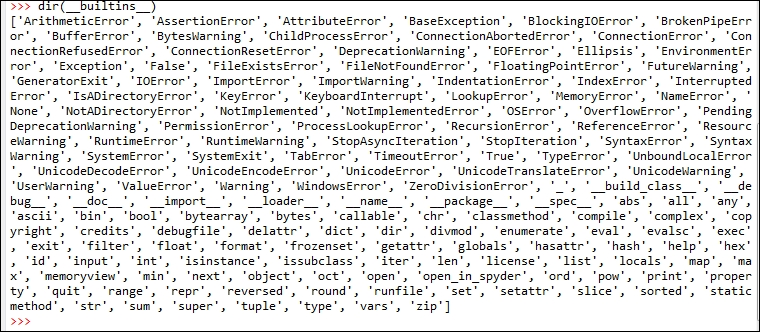## Writing a Python function

Assume that we are interested in writing a Python function for equation (1).

After launching Spyder, click File, then New File. We write the following two lines, as shown in the left panel. The keyword `def` is for function,`fv_f` is the function name, and the three values of `pv`, `r` , and `n` in the pair of parentheses are input variables.

The colon (`:`) indicates the function hasn't finished yet. After we hit the Enter key, the next line will be automatically indented.

After we enter return `pv*(1+r)**n` and hit the Enter key twice, this simple program is completed. Obviously, for the second line, `**` represents a power function.

Assume that we save it under `c:/temp/temp.py`: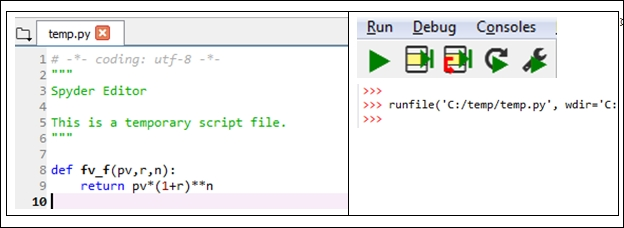To run or debug the program, click the arrow key under Run on the menu bar; see the preceding top-right image. The compiling result is shown by the bottom image right (the second image on top right). Now, we can use this function easily by calling it with three input values:

```>>>fv_f(100,0.1,2)
121.00000000000001
>>>fv_f(100,0.02,20)
148.59473959783548```

If some comments are added by explaining the meanings of input variables, the formula used, plus a few examples, it will be extremely helpful for other users or programmers. Check the following program with comments:

```def pv_f(fv,r,n):
"""Objective: estimate present value
fv
formula  : pv=-------------
(1+r)^n
fv: fture value
r : discount periodic rate
n : number of periods

Example #1  >>>pv_f(100,0.1,1)
90.9090909090909

Example #2: >>>pv_f(r=0.1,fv=100,n=1)
90.9090909090909
"""
return fv/(1+r)**n```

The comments or explanations are included in a pair of three double quotation marks (`"""` and `"""`). The indentation within a comment is not consequential. When compiling, the underlying software will ignore all comments. The beauty of those comments is that we can use `help(pv_f)` to see them, as illustrated here: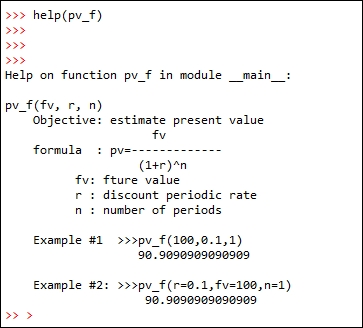In Chapter 2, Introduction to Python Modules, we will show how to upload a financial calculator written in Python, and in Chapter 3, Time Value of Money, we will explain how to generate such a financial calculator.

## Python loops

In this section, we discuss a very important concept: loop or loops. A loop is used to repeat the same task with slightly different input or other factors.

### Python loops, if...else conditions

Let's look at a simple loop through all the data items in an array:

```>>>import numpy as np
>>>cashFlows=np.array([-100,50,40,30])
>>>for cash in cashFlows:
...    print(cash)
...
-100
50
40
30```

One type of data is called a tuple, where we use a pair of parentheses, `()`, to include all input values. One feature of a tuple variable is that we cannot modify its value. This special property could be valuable if some our variables should never be changed.A tuple is different from a dictionary, which stores data with key-value pairs. It is not ordered and it requires that the keys are hashable. Unlike a tuple, the value for a dictionary can be modified.

Note that for Python, the subscription for a vector or tuple starts from `0`. If `x` has a length of `3`, the subscriptions will be `0`, `1` and `2`:

```>>> x=[1,2,3]
>>>x=2
>>>x
>>>
[2, 2, 3]
>>> y=(7,8,9)
>>>y=10
>>>
TypeError: 'tuple' object does not support item assignment
>>>Traceback (most recent call last):
File "<stdin>", line 1, in <module>

>>>type(x)
>>>
<class'list'>
>>>type(y)
>>>
<class'tuple'>
>>>```

Assuming that we invest \$100 today and \$30 next year, the future cash inflow will be \$10, \$40, \$50, \$45, and \$20 at the end of each year for the next 5 years, starting at the end of the second year; see the following timeline and its corresponding cash flows:

```-100    -30       10       40        50         45       20
|--------|---------|--------|---------|----------|--------|
0        1         2        3         4          5        6```

What is the Net Present Value (NPV) if the discount rate is 3.5%? NPVis defined as the present values of all benefits minus the present values of all costs. If a cash inflow has a positive sign while a cash outflow has a negative sign, then NPV can be defined conveniently as the summation of the present values of all cash flows. The present value of one future value is estimated by applying the following formula: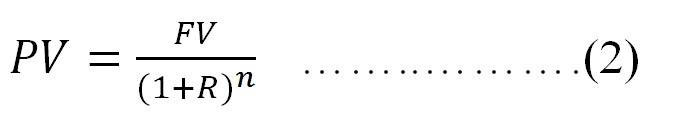Here,PV is the present value, FV is the future value,R is the period discount rate and n is the number of periods. In Chapter 3, Time Value of Money, the meaning of this formula will be explained in more detail. At the moment, we just want to write an`npv_f()` function which applies the preceding equation n times, where n is the number of cash flows. The complete NPV program is given here:

```def npv_f(rate, cashflows):
total = 0.0
for i in range(0,len(cashflows)):
total += cashflows[i] / (1 + rate)**i

In the program, we used a `for` loop. Again, the correct indentation is important for Python. Lines from 2 to 5 are all indented by one unit, thus they belong to the same function, called `npv_f`. Similarly, line 4 is indented two units, that is, after the second column (`:`), it belongs to the `for`loop. The command of `total +=a` is equivalent to `total=total +a`.

For the NPV function, we use a `for` loop. Note that the subscription of a vector in Python starts from zero, and the intermediate variable `i` starts from zero as well. We could call this function easily by entering two sets of input values. The output is shown here:

```>>>r=0.035
>>>cashflows=[-100,-30,10,40,50,45,20]
>>>npv_f(r,cashflows)
14.158224763725372 ```

Here is another `npv_f()` function with a function called `enumerate()`. This function willgenerate a pair of indices, starting from`0`, and its corresponding value:

```def npv_f(rate, cashflows):
total = 0.0
for i, cashflow in enumerate(cashflows):
total += cashflow / (1 + rate)**i

Here is an example illustrating the usage of `enumerate()`:

```x=["a","b","z"]
for i, value in enumerate(x):
print(i, value)```

Unlike the `npv_f` function specified previously, the NPV function from Microsoft Excel is actually a `PV` function, meaning that it can be applied only to the future values. Its equivalent Python program, which is called `npv_Excel`, is shown here:

```def npv_Excel(rate, cashflows):
total = 0.0
for i, cashflow in enumerate(cashflows):
total += cashflow / (1 + rate)**(i+1)

The comparisons are shown in the following table. The result from the Python program is shown in the left panel while the result by calling the Excel NPV function is shown in the right panel. Please pay enough attention to the preceding program shown itself and how to call such a function: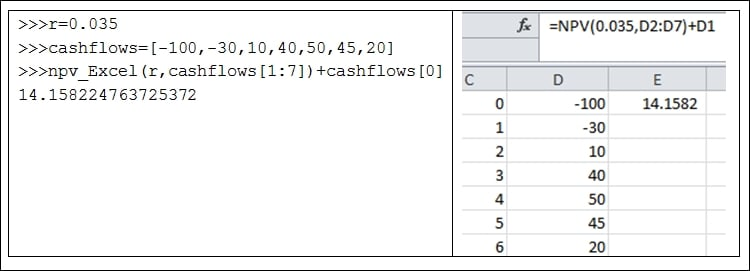By using a loop, we can repeat the same task with different inputs. For example, we plan to print a set of values. The following is such an example for a `while` loop:

```i=1
while(i<10):
print(i)
i+=1```

The following program will report a discount (or any number of discount rates), making its corresponding NPV equal zero. Assume the cash flow will be `550`, `-500`, `-500`, `-500`, and `1000` at time `0`, at the end of each year of the next 4 years. In Chapter 3, Time Value of Money, we will explain the concept of this exercise in more detail.

Write a Python program to find out which discount rate makes NPV equal zero. Since the direction of cash flows changes twice, we might have two different rates making NPV equal zero:

```cashFlows=(550,-500,-500,-500,1000)
r=0
while(r<1.0):
r+=0.000001
npv=npv_f(r,cashFlows)
if(abs(npv)<=0.0001):
print(r)```

The corresponding output is given here:

```0.07163900000005098
0.33673299999790873```

Later in the chapter, a `for`loop is used to estimate the NPV of a project.

When we need to use a few math functions, we can import the `math` module first:

```>>>import math
>>>dir(math)
['__doc__', '__loader__', '__name__', '__package__', '__spec__', 'acos', 'acosh', 'asin', 'asinh', 'atan', 'atan2', 'atanh', 'ceil', 'copysign', 'cos', 'cosh', 'degrees', 'e', 'erf', 'erfc', 'exp', 'expm1', 'fabs', 'factorial', 'floor', 'fmod', 'frexp', 'fsum', 'gamma', 'gcd', 'hypot', 'inf', 'isclose', 'isfinite', 'isinf', 'isnan', 'ldexp', 'lgamma', 'log', 'log10', 'log1p', 'log2', 'modf', 'nan', 'pi', 'pow', 'radians', 'sin', 'sinh', 'sqrt', 'tan', 'tanh', 'trunc']
>>>math.pi
3.141592653589793
>>>```

The `sqrt()`, square root, function is contained in the `math` module. Thus, to use the `sqrt()` function, we need to use `math.sqrt()`; see the following code:

```>>>sqrt(2)
NameError: name 'sqrt' is not defined
>>>Traceback (most recent call last):
File "<stdin>", line 1, in <module>
math.sqrt(2)
1.4142135623730951
>>>```

If we want to call those functions directly, we can use `from math import *`; see the following code:

```>>>from math import *
>>>sqrt(3)
1.7320508075688772
>>>```

To learn about individual embedded functions, we can use the`help()` function;see the following code:

```>>>help(len)
Help on built-in function len in module builtins:
len(obj, /)
Return the number of items in a container.
>>>```

## Data input

Let's generate a very simple input dataset first, as shown here. Its name and location is `c:/temp/test.txt`. The format of the dataset is text:

```a b
1 2
3 4```

The code is shown here:

```>>>f=open("c:/temp/test.txt","r")
>>>f.close()```

The `print()` function could be used to show the value of `x`:

```>>>print(x)
a b
1 2
3 4
>>>```

For the second example, let's download the daily historical price for IBM from Yahoo!Finance first. To do so, we visit http://finance.yahoo.com:Enter `IBM` to find its related web page. Then click Historical Data, then click Download: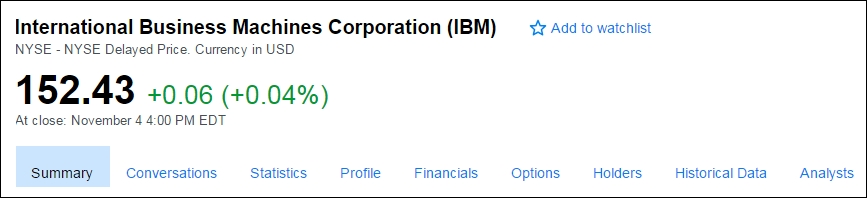Assume that we save the daily data as `ibm.csv` under `c:/temp/`. The first five lines are shown here:

```Date,Open,High,Low,Close,Volume,Adj Close
2016-11-04,152.399994,153.639999,151.869995,152.429993,2440700,152.429993
2016-11-03,152.509995,153.740005,151.800003,152.369995,2878800,152.369995
2016-11-02,152.479996,153.350006,151.669998,151.949997,3074400,151.949997
2016-11-01,153.50,153.910004,151.740005,152.789993,3191900,152.789993```

The first line shows the variable names: date, open price, high price achieved during the trading day, low price achieved during the trading day, close price of the last transaction during the trading day, trading volume, and adjusted price for the trading day. The delimiter is a comma. There are several ways of loading the text file. Some methods are discussed here:

• Method I: We could use `read_csv` from the `pandas` module:

```>>> import pandas as pd
>>>x[1:3]
Date        Open        High         Low       Close   Volume  \
1  2016-11-02  152.479996  153.350006  151.669998  151.949997  3074400
2  2016-11-01  153.500000  153.910004  151.740005  152.789993  3191900

1  151.949997
2  152.789993>>>```
• Method II: We could use `read_table` from the `pandas` module; see the following code:

```>>> import pandas as pd

Alternatively, we could download the IBM daily price data directly from Yahoo!Finance; see the following code:

```>>> import pandas as pd
>>>url=url='http://canisius.edu/~yany/data/ibm.csv'
>>>x[1:5]
Date        Open        High         Low       Close   Volume  \
1  2016-11-03  152.509995  153.740005  151.800003  152.369995  2843600
2  2016-11-02  152.479996  153.350006  151.669998  151.949997  3074400
3  2016-11-01  153.500000  153.910004  151.740005  152.789993  3191900
4  2016-10-31  152.759995  154.330002  152.759995  153.690002  3553200

1  152.369995
2  151.949997
3  152.789993
4  153.690002>>>```

We could retrieve data from an Excel file by using the `ExcelFile()` function from the`pandas` module. First, we generate an Excel file with just a few observations; see the following screenshot: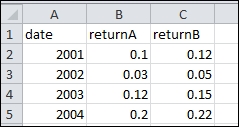Let's call this Excel file `stockReturns.xlxs` and assume that it is saved under `c:/temp/`. The Python code is given here:

```>>>infile=pd.ExcelFile("c:/temp/stockReturns.xlsx")
>>> x=infile.parse("Sheet1")
>>>x
date  returnAreturnB
0  2001     0.10     0.12
1  2002     0.03     0.05
2  2003     0.12     0.15
3  2004     0.20     0.22
>>>```

To retrieve Python datasets with an extension of `.pkl` or `.pickle`, we can use the following code. First, we download the Python dataset called `ffMonthly.pkl` from the author's web page at http://www3.canisius.edu/~yany/python/ffMonthly.pkl.

Assume that the dataset is saved under `c:/temp/`. The function called `read_pickle()` included in the `pandas` module can be used to load the dataset with an extension of `.pkl` or `.pickle`:

```>>> import pandas as pd
>>>x[1:3]
>>>
Mkt_RfSMBHMLRf
196308  0.0507 -0.0085  0.0163  0.0042
196309 -0.0157 -0.0050  0.0019 -0.0080
>>>```

The following is the simplest `if` function: when our interest rate is negative, print a warning message:

```if(r<0):
print("interest rate is less than zero")```

Conditions related to logical `AND` and `OR` are shown here:

```>>>if(a>0 and b>0):
print("both positive")
>>>if(a>0 or b>0):
print("at least one is positive")```

For the multiple `if...elif` conditions, the following program illustrates its application by converting a number grade to a letter grade:

```grade=74
print('A')
print('A-')
print('B+')
print('B')
print('B-')
print('C+')
else:
print('D')```

Note that it is a good idea for such multiple `if...elif` functions to end with an `else` condition since we know exactly what the result is if none of those conditions are met.

## Data manipulation

There are many different types of data, such as integer, real number, or string. The following table offers a list of those data types:

Data types

Description

`Bool`

Boolean (`TRUE` or `FALSE`) stored as a byte

`Int`

Platform integer (normally either `int32` or `int64`)

`int8`

Byte (`-128` to `127`)

`int16`

Integer (`-32768` to `32767`)

`int32`

Integer (`-2147483648` to `2147483647`)

`int64`

Integer (`9223372036854775808` to `9223372036854775807`)

`unit8`

Unsigned integer (`0` to `255`)

`unit16`

Unsigned integer (`0` to `65535`)

`unit32`

Unsigned integer (`0` to `4294967295`)

`unit64`

Unsigned integer (`0` to `18446744073709551615`)

`float`

Short and for `float6`

`float32`

Single precision float: sign `bit23` bits mantissa; 8 bits exponent

`float64`

52 bits mantissa

`complex`

Shorthand for `complex128`

`complex64`

Complex number; represented by two 32-bit floats (real and imaginary components)

`complex128`

Complex number; represented by two 64-bit floats (real and imaginary components)

Table 1.1 List of different data types

In the following examples, we assign a value to `r`, which is a scalar, and several values to `pv`, which is an array (vector).The `type()` function is used to show their types:

```>>> import numpy as np
>>> r=0.023
>>>pv=np.array([100,300,500])
>>>type(r)
<class'float'>
>>>type(pv)
<class'numpy.ndarray'>```

To choose the appropriate decision, we use the `round()`function; see the following example:

```>>> 7/3
2.3333333333333335
>>>round(7/3,5)
2.33333
>>>```

For data manipulation, let's look at some simple operations:

```>>>import numpy as np
>>>a=np.zeros(10)                      # array with 10 zeros
>>>b=np.zeros((3,2),dtype=float)       # 3 by 2 with zeros
>>>c=np.ones((4,3),float)              # 4 by 3 with all ones
>>>d=np.array(range(10),float)         # 0,1, 2,3 .. up to 9
>>>e1=np.identity(4)                   # identity 4 by 4 matrix
>>>e2=np.eye(4)                        # same as above
>>>e3=np.eye(4,k=1)                    # 1 start from k
>>>f=np.arange(1,20,3,float)           # from 1 to 19 interval 3
>>>g=np.array([[2,2,2],[3,3,3]])       # 2 by 3
>>>h=np.zeros_like(g)                  # all zeros
>>>i=np.ones_like(g)                   # all ones```

Some so-called `dot` functions are quite handy and useful:

```>>> import numpy as np
>>> x=np.array([10,20,30])
>>>x.sum()
60```

Anything after the number sign of `#` will be a comment. Arrays are another important data type:

```>>>import numpy as np
>>>x=np.array([[1,2],[5,6],[7,9]])      # a 3 by 2 array
>>>y=x.flatten()
>>>x2=np.reshape(y,[2,3]              ) # a 2 by 3 array```

We could assign a string to a variable:

```>>> t="This is great"
>>>t.upper()
'THIS IS GREAT'
>>>```

To find out all string-related functions, we use `dir('')`; see the following code:

```>>>dir('')
['__add__', '__class__', '__contains__', '__delattr__', '__dir__', '__doc__', '__eq__', '__format__', '__ge__', '__getattribute__', '__getitem__', '__getnewargs__', '__gt__', '__hash__', '__init__', '__iter__', '__le__', '__len__', '__lt__', '__mod__', '__mul__', '__ne__', '__new__', '__reduce__', '__reduce_ex__', '__repr__', '__rmod__', '__rmul__', '__setattr__', '__sizeof__', '__str__', '__subclasshook__', 'capitalize', 'casefold', 'center', 'count', 'encode', 'endswith', 'expandtabs', 'find', 'format', 'format_map', 'index', 'isalnum', 'isalpha', 'isdecimal', 'isdigit', 'isidentifier', 'islower', 'isnumeric', 'isprintable', 'isspace', 'istitle', 'isupper', 'join', 'ljust', 'lower', 'lstrip', 'maketrans', 'partition', 'replace', 'rfind', 'rindex', 'rjust', 'rpartition', 'rsplit', 'rstrip', 'split', 'splitlines', 'startswith', 'strip', 'swapcase', 'title', 'translate', 'upper', 'zfill']
>>>```

For example, from the preceding list we see a function called `split`. After typing`help(''.split)`, we will have related help information:

```>>>help(''.split)
Help on built-in function split:

split(...) method of builtins.str instance
S.split(sep=None, maxsplit=-1) -> list of strings

Return a list of the words in S, using sep as the
delimiter string. If maxsplit is given, at most maxsplit
splits are done. If sep is not specified or is None, any
whitespace string is a separator and empty strings are
removed from the result.
>>>```

We could try the following example:

```>>> x="this is great"
>>>x.split()
['this', 'is', 'great']
>>>```

Matrix manipulation is important when we deal with various matrices: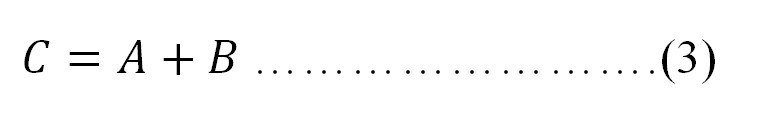The condition for equation (3) is that matrices A and B should have the same dimensions. For the product of two matrices, we have the following equation: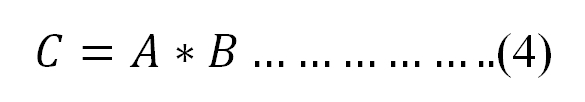Here,A is an n by k matrix (n rows and k columns), while B is a k by m matrix. Remember that the second dimension of the first matrix should be the same as the first dimension of the second matrix. In this case, it is k. If we assume that the individual data items in C, A, and B are Ci,j (the ith row and the jth column), Ai,j, and Bi,j, we have the following relationship between them: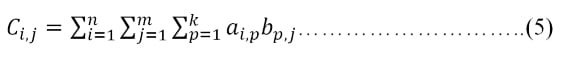The `dot()` function from the NumPy module could be used to carry the preceding matrix multiplication:

```>>>a=np.array([[1,2,3],[4,5,6]],float)    # 2 by 3
>>>b=np.array([[1,2],[3,3],[4,5]],float)  # 3 by 2
>>>np.dot(a,b)                            # 2 by 2
>>>print(np.dot(a,b))
array([[ 19.,  23.],
[ 43.,  53.]])
>>>```

We could manually calculate c(1,1): 1*1 + 2*3 + 3*4=19.

After retrieving data or downloading data from the internet, we need to process it. Such a skill to process various types of raw data is vital to finance students and to professionals working in the finance industry. Here we will see how to download price data and then estimate returns.

Assume that we have n values of x1, x2, … and xn. There exist two types of means: arithmetic mean and geometric mean; see their genetic definitions here: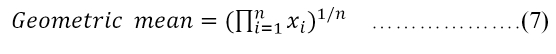Assume that there exist three values of `2`,`3`, and `4`. Their arithmetic and geometric means are calculated here:

```>>>(2+3+4)/3.
>>>3.0
>>>geo_mean=(2*3*4)**(1./3)
>>>round(geo_mean,4)
2.8845```

For returns, the arithmetic mean's definition remains the same, while the geometric mean of returns is defined differently; see the following equations: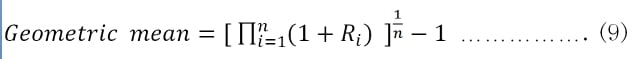In Chapter 3, Time Value of Money, we will discuss both means again.

We could say that NumPy is a basic module while SciPy is a more advanced one. NumPy tries to retain all features supported by either of its predecessors, while most new features belong in SciPy rather than NumPy. On the other hand, NumPy and SciPy have many overlapping features in terms of functions for finance. For those two types of definitions, see the following example:

```>>> import scipy as sp
>>> ret=sp.array([0.1,0.05,-0.02])
>>>sp.mean(ret)
0.043333333333333342
>>>pow(sp.prod(ret+1),1./len(ret))-1
0.042163887067679262```

Our second example is related to processing theFama-French 3 factor time series. Since this example is more complex than the previous one, if a user feels it is difficult to understand, he/she could simply skip this example. First, a ZIP file called `F-F_Research_Data_Factor_TXT.zip` could be downloaded from Prof. French's Data Library. After unzipping and removing the first few lines and annual datasets, we will have a monthly Fama-French factor time series. The first few lines and last few lines are shown here:

```DATE    MKT_RFSMBHMLRF
192607    2.96   -2.30   -2.87    0.22
192608    2.64   -1.40    4.19    0.25
192609    0.36   -1.32    0.01    0.23

201607    3.95    2.90   -0.98    0.02
201608    0.49    0.94    3.18    0.02
201609    0.25    2.00   -1.34    0.02```

Assume that the final file is called `ffMonthly.txt` under `c:/temp/`. The following program is used to retrieve and process the data:

```import numpy as np
import pandas as pd
file=open("c:/temp/ffMonthly.txt","r")
f=[]
index=[]
for i in range(1,np.size(data)):
t=data[i].split()
index.append(int(t))
for j in range(1,5):
k=float(t[j])
f.append(k/100)
n=len(f)
f1=np.reshape(f,[n/4,4])
ff=pd.DataFrame(f1,index=index,columns=['Mkt_Rf','SMB','HML','Rf'])```

To view the first and last few observations for the dataset called `ff`, the functions of `.head()` and `.tail()`can be used: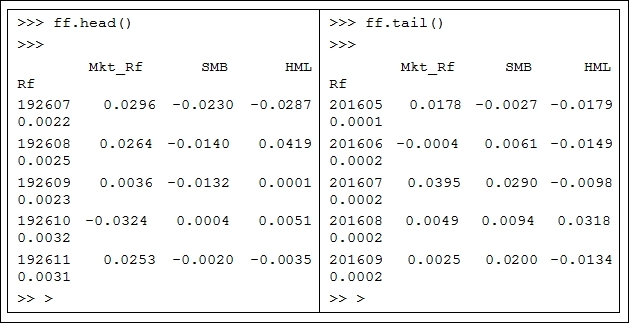## Data output

The simplest example is given here:

```>>>f=open("c:/temp/out.txt","w")
>>>x="This is great"
>>>f.write(x)
>>>f.close()```

For the next example, we download historical stock price data first, then write data to an output file:

```import re
from matplotlib.finance import quotes_historical_yahoo_ochl
ticker='dell'
outfile=open("c:/temp/dell.txt","w")
begdate=(2013,1,1)
enddate=(2016,11,9)
p=quotes_historical_yahoo_ochl
outfile.write(str(p))
outfile.close()```

To retrieve the file, we have the following code:

```>>>infile=open("c:/temp/dell.txt","r")

One issue is that the preceding saved text file contains many unnecessary characters, such as `[` and`]`. We could apply a substitution function called `sub()` contained in the Python module;see the simplest example given here:

```>>> import re
>>>re.sub("a","9","abc")
>>>
'9bc'
>>>```

In the preceding example, we will replace the letter `a` with`9`. Interested readers could try the following two lines of code for the preceding program:

```p2= re.sub('[\(\)\{\}\.<>a-zA-Z]','', p)
outfile.write(p2)```

It is a good idea to generate Python datasets with an extension of `.pickle` since we can retrieve such data quite efficiently. The following is the complete Python code to generate `ffMonthly.pickle`. Here, we show how to download price data and then estimate returns:

```import numpy as np
import pandas as pd
file=open("c:/temp/ffMonthly.txt","r")
f=[]
index=[]
for i in range(1,np.size(data)):
t=data[i].split()
index.append(int(t))
for j in range(1,5):
k=float(t[j])
f.append(k/100)
n=len(f)
f1=np.reshape(f,[n/4,4])
ff=pd.DataFrame(f1,index=index,columns=['Mkt_Rf','SMB','HML','Rf'])
ff.to_pickle("c:/temp/ffMonthly.pickle")```

## Exercises

2. Is Python case-sensitive?

3. How do you assign a set of values to pv in the format of a tuple. Could we change its values after the assignment?

4. Estimate the area of a circle if the diameter is 9.7 using Python.

5. How do you assign a value to a new variable?

6. How can you find some sample examples related to Python?

7. How do you launch Python's help function?

8. How can you find out more information about a specific function, such as `print()`?

9. What is the definition of built-in functions?

10. Is `pow()` a built-in function? How do we use it?

11. How do we find all built-in functions? How many built-in functions are present?

12. When we estimate the square root of 3, which Python function should we use?

13. Assume that the present value of a perpetuity is \$124 and the annual cash flow is \$50; what is the corresponding discount rate? The formula is given here: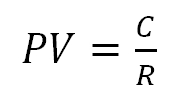14. Based on the solution of the previous question, what is the corresponding quarterly rate?

15. For a perpetuity, the same cash flow happens at the same interval forever. A growing perpetuity is defined as follows: the future cash flow is increased at a constant growth rate forever. If the first cash flow happens at the end of the first period, we have the following formula: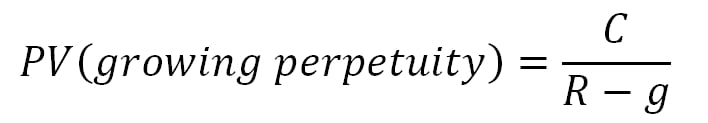Here PV is the present value, C is the cash flow of the next period, g is a growth rate, and R is the discount rate. If the first cash flow is \$12.50, the constant growth rate is 2.5 percent, and the discount rate is 8.5 percent. What is the present value of this growing perpetuity?

16. For an n-day variance, we have the following formula: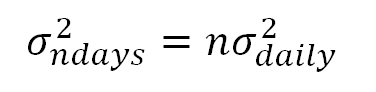Here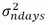is the daily variance and is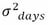is the daily standard deviation (volatility). If the volatility (daily standard deviation) of a stock is 0.2, what is its 10-day volatility?

17. We expect to have \$25,000 in 5 years. If the annual deposit rate is 4.5 percent, how much do we have to deposit today?

18. The substitution function called `sub()` is from a Python module. Find out how many functions are contained in that module.

19. Write a Python program to convert the standard deviation estimated based on daily data or monthly data to an annual one by using the following formulas: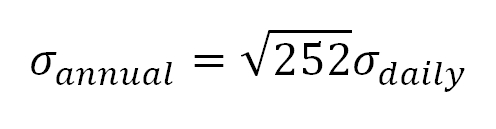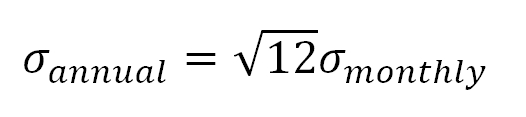20. The Sharpe ratio is a measure of trade-off between benefit (excess return) and cost (total risk) for an investment such as a portfolio. Write a Python program to estimate the Sharpe ratio by applying the following formula: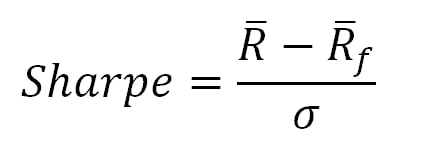Here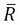is the portfolio mean return,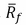is the mean risk-free rate and σ is the risk of the portfolio. Again, at this moment, it is perfectly fine that a reader does not understand the economic meaning of this ratio since the Sharpe ratio will be discussed in more detail in Chapter 7, Multifactor Models and Performance Measures.

## Summary

In this chapter, many basic concepts and several widely used functions related to Python werediscussed. In Chapter 2, Introduction to Python Modules, we will discuss a key component of the Python language: Python modules and theirrelated issues. A module is a set of programs written by experts, professionals, or any person around a specific topic. A module could be viewed as a toolbox for a specific task. The chapter willfocus on the five most important modules: NumPy, SciPy, `matplotlib`, `statsmodels`, and `pandas`.

• ##### Yuxing Yan

Yuxing Yan graduated from McGill University with a PhD in finance. Over the years, he has been teaching various finance courses at eight universities: McGill University and Wilfrid Laurier University (in Canada), Nanyang Technological University (in Singapore), Loyola University of Maryland, UMUC, Hofstra University, University at Buffalo, and Canisius College (in the US).

His research and teaching areas include: market microstructure, open-source finance and financial data analytics. He has 22 publications including papers published in the Journal of Accounting and Finance, Journal of Banking and Finance, Journal of Empirical Finance, Real Estate Review, Pacific Basin Finance Journal, Applied Financial Economics, and Annals of Operations Research.

He is good at several computer languages, such as SAS, R, Python, Matlab, and C.

His four books are related to applying two pieces of open-source software to finance: Python for Finance (2014), Python for Finance (2nd ed., expected 2017), Python for Finance (Chinese version, expected 2017), and Financial Modeling Using R (2016).

In addition, he is an expert on data, especially on financial databases. From 2003 to 2010, he worked at Wharton School as a consultant, helping researchers with their programs and data issues. In 2007, he published a book titled Financial Databases (with S.W. Zhu). This book is written in Chinese.

Currently, he is writing a new book called Financial Modeling Using Excel — in an R-Assisted Learning Environment. The phrase "R-Assisted" distinguishes it from other similar books related to Excel and financial modeling. New features include using a huge amount of public data related to economics, finance, and accounting; an efficient way to retrieve data: 3 seconds for each time series; a free financial calculator, showing 50 financial formulas instantly, 300 websites, 100 YouTube videos, 80 references, paperless for homework, midterms, and final exams; easy to extend for instructors; and especially, no need to learn R.

Browse publications by this author

### Latest Reviews

(5 reviews total)